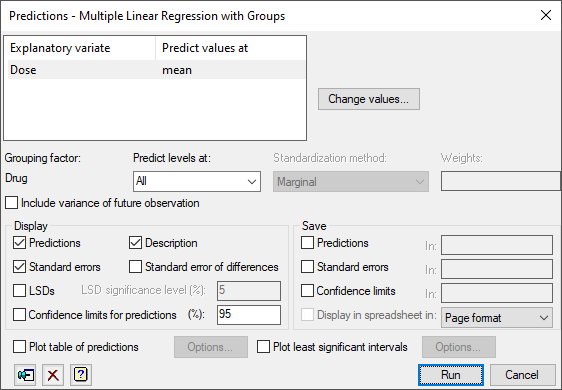1. Home
2. Predictions from Multiple Linear Regression (Grouped)

# Predictions from Multiple Linear Regression (Grouped)

Use this to predict the value for a future observation based on the current fitted linear regression model.## List of explanatory variates

Lists all the explanatory variables in the current regression model along with the value to predict the response variable from. You can change the values to predict the response variable from by either double-clicking on an explanatory variable name or by selecting the names and clicking on the Change values button.

 Change values Opens a window that lets you change the values of the currently selected explanatory variables.

## Grouping factor

Displays the name of the grouping factor for which the values of the response variable are to be predicted from.

## Predict levels at

Specify the grouping levels at which the predictions for the response variable are to be calculated. You can either type one or more of the levels in directly, or select a method from the dropdown list.

## Standardization method

Allows you standardize the table of predictions. The Marginal option standardizes the table of predictions by weighting by the number of observations within each level of the factor(s). Equal standardizes by having equal weight for each of the levels of the factor(s). The Specify option lets you explicitly specify the weightings to be used in the predictions by entering the weights in the space provided.

## Weights

Enter the weights when the Specify option is selected for the standardization method.

## Include variance of future observation

Specifies whether you want the variance of predictions to be calculated on the basis of forecasting new observations. The default is for the variance of predictions to be calculated on the basis of summarizing the data to which the data has been fitted.

## Display

Specifies which items of output are to be produced.

 Predictions Predictions Description Describes the standardization policies used when forming predictions Standard errors Standard errors of predictions Standard error of differences Standard error of differences between predictions LSDs Least significant differences between predictions (for ordinary linear regression models or GLMs with Normal distribution only). The LSD significance level (%) can be specified in the space provided. Confidence limits for predictions Confidence limits for each prediction. The confidence level (%) can be specified in the space provided.

## Save

 Predictions Table or scalar Predicted values for each response value Standard errors Table or scalar Standard errors for each predicted value Confidence limits Table or scalar Confidence limits for each predicted value. The confidence level is that set in the Display section.

Display the saved results in a new spreadsheet window.

## Plot table of predictions

If this is selected, the predictions will be plotted. The Options button opens the Plot table of predictions dialog which controls how the predictions are plotted. You need to complete either the Grouping factor or Explanatory variates before using the Options button.

## Plot least significant intervals

If this is selected, the least significant intervals for the means will be plotted. Least significant intervals (LSIs) are used for comparing a set of predicted means graphically, especially when their SEDs differ. LSIs are intervals (or error bars) that are designed to overlap where there is no significant difference between estimates, and to be disjoint (i.e. not to overlap) where there are significant differences. The Options button opens the Least Significant Intervals Plot Options dialog which controls how the least significant intervals are plotted. There must be more than one estimated mean for a graph to be produced.